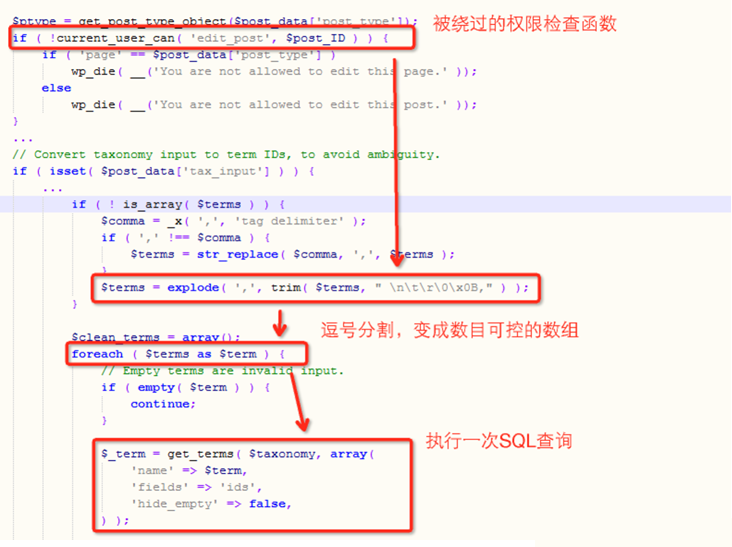### WordPress SQL注入漏洞与提权分析

WordPress核心功能SQL注入漏洞分析

## 0x01 越权提交文章

### _wpnonce的获取

```case 'post-quickdraft-save':
// Check nonce and capabilities
\$nonce = \$_REQUEST['_wpnonce'];
\$error_msg = false;

// For output of the quickdraft dashboard widget

if ( ! wp_verify_nonce( \$nonce, 'add-post' ) )
\$error_msg = __( 'Unable to submit this form, please refresh and try again.' );

if ( ! current_user_can( 'edit_posts' ) )

if ( \$error_msg )
return wp_dashboard_quick_press( \$error_msg );
```

```wp_nonce_field( 'add-post' );
```

### 越权提交与条件竞争

```if ( isset( \$_GET['post'] ) )
\$post_id = \$post_ID = (int) \$_GET['post'];
elseif ( isset( \$_POST['post_ID'] ) )
\$post_id = \$post_ID = (int) \$_POST['post_ID'];
else
\$post_id = \$post_ID = 0;

global \$post_type, \$post_type_object, \$post;

if ( \$post_id )
\$post = get_post( \$post_id );

if ( \$post ) {
\$post_type = \$post->post_type;
\$post_type_object = get_post_type_object( \$post_type );
}

```

```function edit_post( \$post_data = null ) {
global \$wpdb;

if ( empty(\$post_data) )
\$post_data = &\$_POST;

// Clear out any data in internal vars.
unset( \$post_data['filter'] );

\$post_ID = (int) \$post_data['post_ID'];
\$post = get_post( \$post_ID );
\$post_data['post_type'] = \$post->post_type;
\$post_data['post_mime_type'] = \$post->post_mime_type;

if ( ! empty( \$post_data['post_status'] ) ) {
\$post_data['post_status'] = sanitize_key( \$post_data['post_status'] );

if ( 'inherit' == \$post_data['post_status'] ) {
unset( \$post_data['post_status'] );
}
}

\$ptype = get_post_type_object(\$post_data['post_type']);
if ( !current_user_can( 'edit_post', \$post_ID ) ) {
if ( 'page' == \$post_data['post_type'] )
else
wp_die( __('You are not allowed to edit this post.' ));
}
```

```case 'edit_post':
case 'edit_page':
\$post = get_post( \$args );
if ( empty( \$post ) )
break;

if ( 'revision' == \$post->post_type ) {
\$post = get_post( \$post->post_parent );
}
```

```public function has_cap( \$cap ) {
if ( is_numeric( \$cap ) ) {
_deprecated_argument( __FUNCTION__, '2.0', __('Usage of user levels by plugins and themes is deprecated. Use roles and capabilities instead.') );
\$cap = \$this->translate_level_to_cap( \$cap );
}

\$args = array_slice( func_get_args(), 1 );
\$args = array_merge( array( \$cap, \$this->ID ), \$args );
\$caps = call_user_func_array( 'map_meta_cap', \$args );

// Multisite super admin has all caps by definition, Unless specifically denied.
if ( is_multisite() && is_super_admin( \$this->ID ) ) {
if ( in_array('do_not_allow', \$caps) )
return false;
return true;
}

\$capabilities = apply_filters( 'user_has_cap', \$this->allcaps, \$caps, \$args, \$this );
\$capabilities['exist'] = true; // Everyone is allowed to exist
foreach ( (array) \$caps as \$cap ) {
if ( empty( \$capabilities[ \$cap ] ) )
return false;
}

return true;
}
```

```if ( isset( \$post_data['tax_input'] ) ) {
foreach ( (array) \$post_data['tax_input'] as \$taxonomy => \$terms ) {
// Hierarchical taxonomy data is already sent as term IDs, so no conversion is necessary.
if ( is_taxonomy_hierarchical( \$taxonomy ) ) {
continue;
}

if ( ! is_array( \$terms ) ) {
\$comma = _x( ',', 'tag delimiter' );
if ( ',' !== \$comma ) {
\$terms = str_replace( \$comma, ',', \$terms );
}
\$terms = explode( ',', trim( \$terms, " \n\t\r\x0B," ) );
}

\$clean_terms = array();
foreach ( \$terms as \$term ) {
// Empty terms are invalid input.
if ( empty( \$term ) ) {
continue;
}

\$_term = get_terms( \$taxonomy, array(
'name' => \$term,
'fields' => 'ids',
'hide_empty' => false,
) );
```## 0x02 SQL注入漏洞

### revision trick

revisions字段用于记录草稿或者更新的发布，Wordpress中用revisions记录对于完成的提交和存储都会在posts数据库表中将post_type设置成revision，每个revision都会有一个’post_parent’字段，指明这个revision所基于的原版提交。

### 漏洞原因

```function wp_untrash_post_comments( \$post = null ) {
global \$wpdb;

\$post = get_post(\$post);
if ( empty(\$post) )
return;

\$post_id = \$post->ID;

if ( empty(\$statuses) )
return true;

// Restore each comment to its original status.
\$group_by_status = array();
foreach ( \$statuses as \$comment_id => \$comment_status )
\$group_by_status[\$comment_status][] = \$comment_id;

foreach ( \$group_by_status as \$status => \$comments ) {
// Sanity check. This shouldn't happen.
if ( 'post-trashed' == \$status )
\$status = '0';
\$wpdb->query( "UPDATE \$wpdb->comments SET comment_approved = '\$status' WHERE comment_ID IN ('" . \$comments_in . "')" );
}

clean_comment_cache( array_keys(\$statuses) );

}

```

### 漏洞利用&对作者深深的怨念

1. 对写入文章的revision进行评论
2. 编辑评论状态为注入攻击语句
3. 将revision丢入垃圾箱
4. 还原最开始的文章（revision的原版）

## 0x03 总结

GP操作的逻辑是Web代码的一个问题，尤其是在做一些权限校验时候，很容易出现Wordpress这样的GP交叉导致的逻辑混乱问题。

## 0x04 参考文章

【3】Wordpress4.2.3提权与SQL注入漏洞(CVE-2015-5623)分析